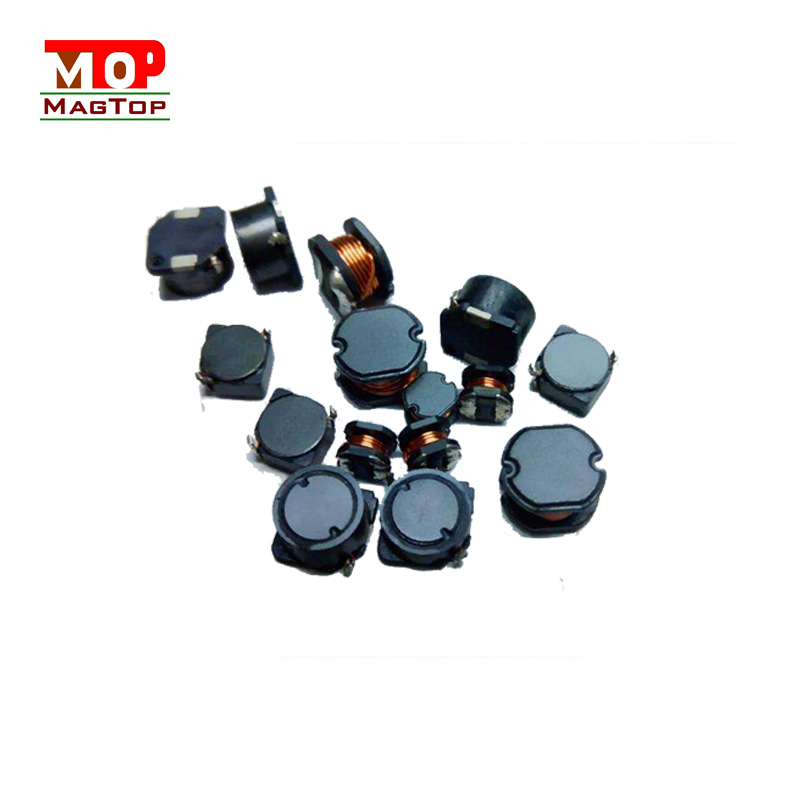# What factors are related to the inductance of the inductor

##### author: MagTop
11/07/2022The parameters of the inductance are very important for the function of the inductor. It also has many parameters, such as inductance, current, standard, etc., which play an important role in the selection of the inductance and the application environment. This article mainly discusses the relationship between the size of the magnetic ring inductance and the number of coil turns, the difference between the manganese-zinc magnetic ring inductance and the nickel-zinc-ferromagnetic ring inductance, which will involve an inductance parameter - inductance. Today I will share with you the inductance What are the factors related to the size of the amount.
To understand what factors are related to the magnitude of inductive induction, it is necessary to understand what an inductance is. An electrical inductor is simply the passing of an alternating current in a wire, generating an alternating magnetic flux in and around the wire, the ratio of the magnetic flux of the wire to the current that produces it. Below is the definition of inductance.
A parameter, related to the number of turns, size and medium of the coil, that is a measure of the inertia of the induction coil, independent of the applied current.

The induction coil is also an accumulator, which stores electrical energy magnetically. Generally, the greater the inductance of the coil, the greater the current flowing through it.

The large size of electromagnetic field induction is related to coil diameter, number of turns, enameled wire diameter, winding density, etc. Capacitance is a characteristic of the coil itself and has nothing to do with the magnitude of the current.